# Multiplication strategies#### Everything You Need in One Place

Homework problems? Exam preparation? Trying to grasp a concept or just brushing up the basics? Our extensive help & practice library have got you covered.#### Learn and Practice With Ease

Our proven video lessons ease you through problems quickly, and you get tonnes of friendly practice on questions that trip students up on tests and finals.#### Instant and Unlimited Help

Our personalized learning platform enables you to instantly find the exact walkthrough to your specific type of question. Activate unlimited help now!

0/6
##### Intros
###### Lessons
1. Introduction to Multiplication Strategies:
2. Using addition and arrays to understand multiplication
3. Breaking down multiplication facts into smaller groupsr
4. A product can be found using a smaller product and a sum
5. A product can be found using a bigger product and a difference
6. Patterns to know for memorizing multiples of 9
7. Finger method of 9 times tables
0/13
##### Examples
###### Lessons
1. Understanding products using smaller products
Turn the product into the sum of two smaller group products.
1. 8 × 4 = (5 × 4) + ( __ × 4)
$\qquad \qquad$ = _____ + _____
$\qquad \qquad$ = _______
2. 12 × 12 = (10 × 12) + ( __ × 12)
$\qquad \qquad$ = _______ + _______
$\qquad \qquad$ = _______
3. 20 × 35 = (10 × 35) + ( __ × 35)
$\qquad \qquad$ = _______ + _______
$\qquad \qquad$ = _______
2. Describing multiplication array models - 1
Fill in the blanks to describe:
1. the product shown in the array and
2. the sum written with the smaller product

1.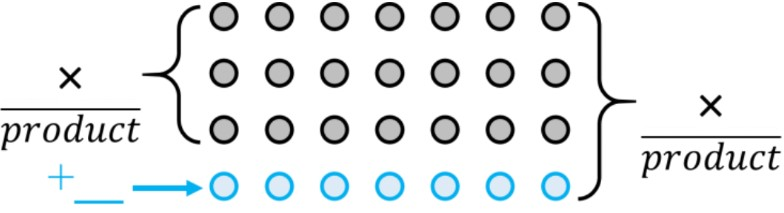2.3. Describing multiplication array models - 2
Fill in the blanks to turn the product into a smaller product and sum.
Use an array model to help fill in the blanks.
1. 5 × 3 = ( __ × 3) + 3
$\qquad \qquad$ = _______ + 3
$\qquad \qquad$ = _______
2. 11 × 12 = ( __ × 12) + __
$\qquad \qquad$ = _______ + ____
$\qquad \qquad$ = _______
4. Multiplication and array models with subtraction
Fill in the blanks to turn the product into a bigger product and a difference.
1. 9 × 6 = ( __ × 6) - 6
$\qquad \qquad$ = _______ + ____
$\qquad \qquad$ = _______
2. 9 × 27 = ( __ × 27) - 27
$\qquad \qquad$ = _______ - ____
$\qquad \qquad$ = _______
5. Relating multiplication and addition concepts
Find the answer using the given product.
1. If 6 × 86 = 516 , what is 7 × 86 = ?
2. If 10 × 53 = 530 , what is 9 × 53 = ?
6. Multiplication with 9-times tables strategy: word problems
Use the finger method for 9-times tables to solve.
1. If you put down the fourth finger, what is the 9-times tables multiplication sentence that is represented?
2. If you put down the fourth finger, it represents a 9-times table fact. What finger do you need to put down for the opposite answer (when the answer's digits are flipped/mirrored)? Write the multiplication sentence for the 9-times table fact with the opposite answer
0%
##### Practice
###### Free to Join!
StudyPug is a learning help platform covering math and science from grade 4 all the way to second year university. Our video tutorials, unlimited practice problems, and step-by-step explanations provide you or your child with all the help you need to master concepts. On top of that, it's fun - with achievements, customizable avatars, and awards to keep you motivated.
• #### Easily See Your ProgressWe track the progress you've made on a topic so you know what you've done. From the course view you can easily see what topics have what and the progress you've made on them. Fill the rings to completely master that section or mouse over the icon to see more details.
• #### Make Use of Our Learning Aids###### Practice Accuracy

Get quick access to the topic you're currently learning.

See how well your practice sessions are going over time.

Stay on track with our daily recommendations.

• #### Earn Achievements as You LearnMake the most of your time as you use StudyPug to help you achieve your goals. Earn fun little badges the more you watch, practice, and use our service.
• #### Create and Customize Your AvatarPlay with our fun little avatar builder to create and customize your own avatar on StudyPug. Choose your face, eye colour, hair colour and style, and background. Unlock more options the more you use StudyPug.
###### Topic Notes

In this lesson, we will learn:

• Understanding multiplication using arrays
• Representing a product as either: (1) a smaller product and a sum, or (2) a bigger product and a difference
• Tips and tricks for memorizing the 9 × multiplication table facts

Notes:

• Multiplication is just repeated addition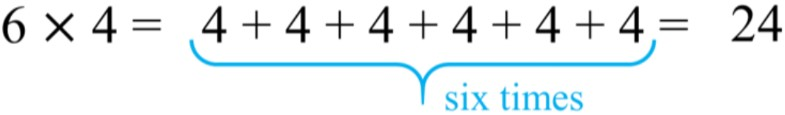• Multiplication facts can be shown in an array model with circles/dots
• Using the array model, it shows that multiplication facts can be broken into groups of smaller multiplication facts: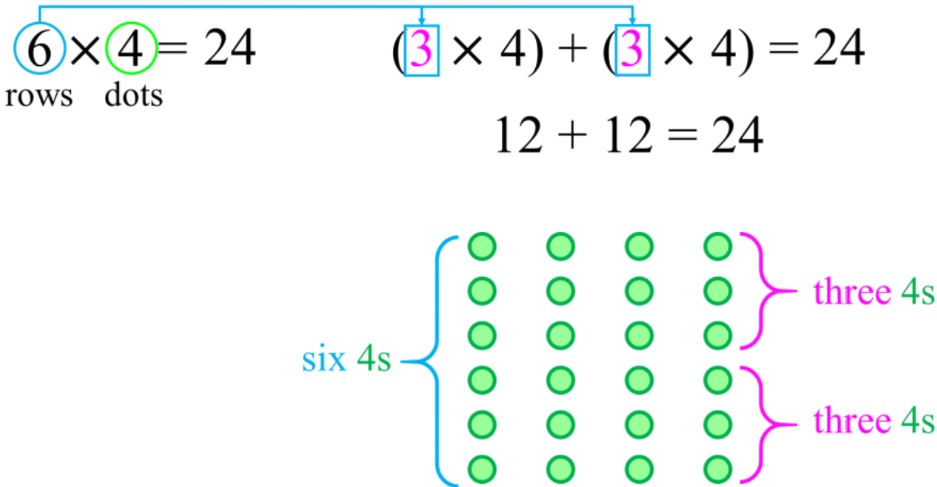• Using the same array model, we can find the next multiplication fact by adding another row: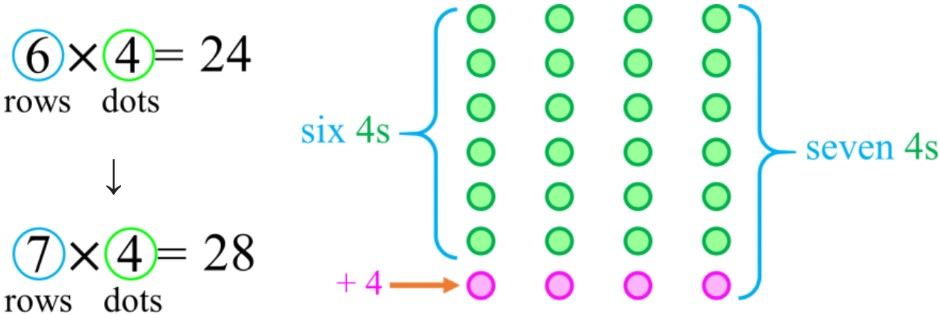• Therefore, a product can be found as a smaller product and a sum• Or, it could be found as a bigger product and a difference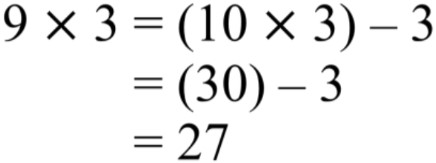• The 9 × multiplication tables can be memorized using your fingers!
• Notice that the first ten multiples of 9 are mirrored after the 5-digit in 45
• Ex. 9, 18, 27, 36, 45 $\parallel$ 54, 63, 72, 81, 90